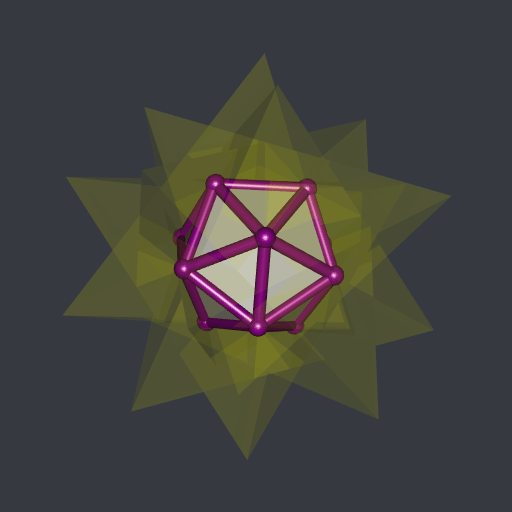# Boolean operations on meshes with R(CGAL)

Posted on May 14, 2022 by Stéphane Laurent
Tags: geometry, R, rgl, graphics, C++

I’m still working on my package RCGAL, that I already present in a previous post.

This previous post was about the reconstruction of surfaces. Now I implemented the Boolean operations on meshes. Here are some simple examples.

#### Union of two cubes: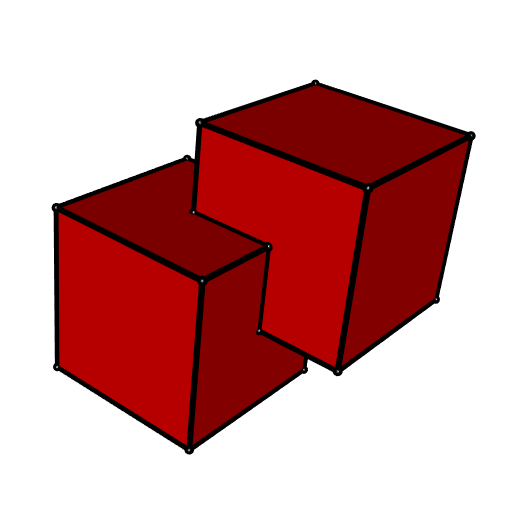#### Intersection of two cubes (one rotated):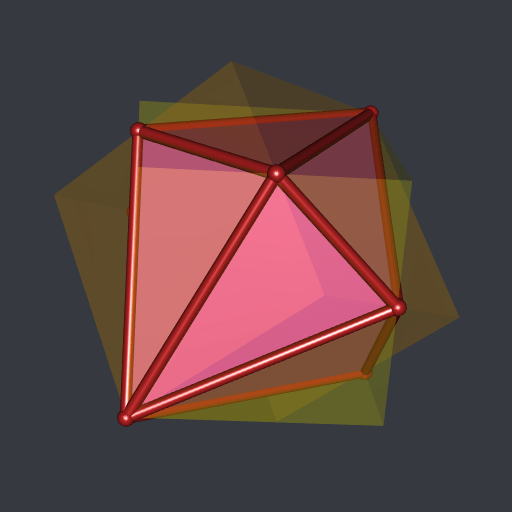#### Difference of two cubes: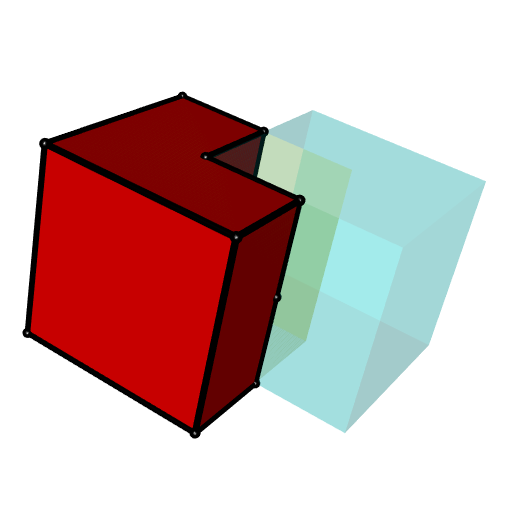#### Intersection of a cube and a truncated icosahedron: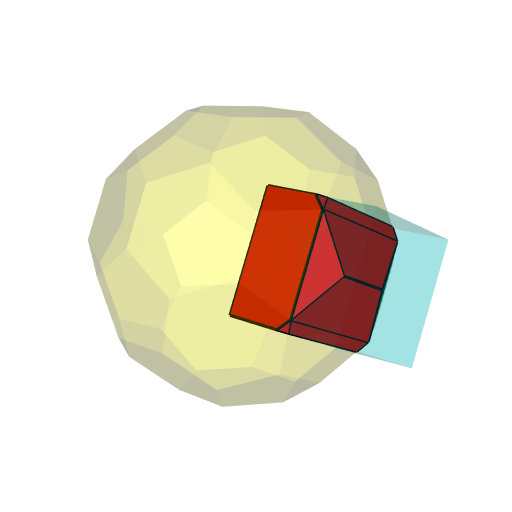The code generating these plots is given in the RCGAL examples.

Now let’s turn to a more interesting example.

## The compound of five tetrahedra

The compound of five tetrahedra is provided by RCGAL. These are five tetrahedra in a pretty configuration, each centered at the origin. You can get their meshes by typing tetrahedraCompound. This is a list with two components: a field meshes providing for each tetrahderon its vertices and its faces, and a field rglmeshes, similar to meshes but these meshes are ready for plotting with the rgl package. Here it is:

library(RCGAL)
library(rgl)
rglmeshes <- tetrahedraCompound[["rglmeshes"]]
open3d(windowRect = c(50, 50, 562, 562), zoom = 0.75)
bg3d("#363940")
colors <- hcl.colors(5, palette = "Spectral")
invisible(lapply(
1:5, function(i) shade3d(rglmeshes[[i]], color = colors[i])
))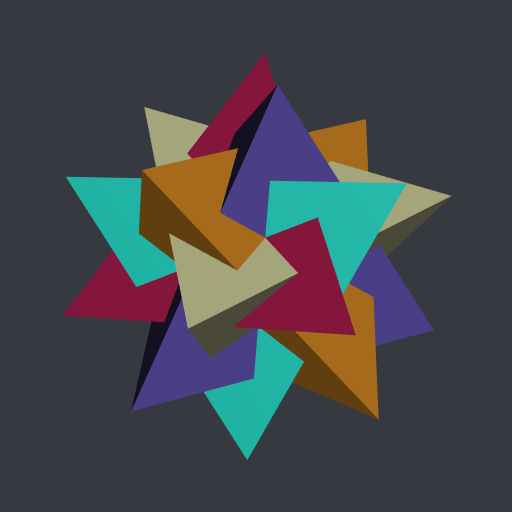I wondered for a long time what is the intersection of these five tetrahedra. But I didn’t have any tool to compute it. Now I have. Let’s see.

# compute the intersection ####
inter <- MeshesIntersection(
tetrahedraCompound[["meshes"]], numbersType = "lazyExact", clean = TRUE
)
# plot ####
open3d(windowRect = c(50, 50, 562, 562), zoom = 0.75)
bg3d("#363940")
# first the five tetrahedra with transparency ####
invisible(lapply(
rglmeshes, shade3d, color = "yellow", alpha = 0.1
))
# now the intersection ####
rglinter <- tmesh3d(
"vertices"    = t(inter[["vertices"]]),
"indices"     = t(inter[["faces"]]),
"homogeneous" = FALSE
)
)